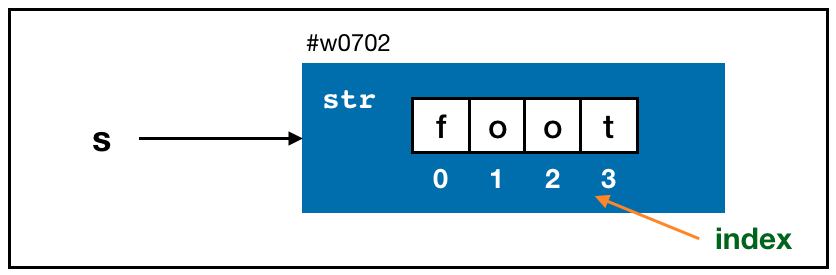## Scalar Types

• Scalar refers to “single value” type of data.
• Numerical data (integer or float), strings, boolean (True or False) values.
• The table below contains all standard Python scalar types.
Type Description
None The Python “null” value
str String type, holds Unicode (UTF-8 encoded) strings
bytes Raw ASCII bytes (or Unicode encoded as bytes)
float Double-precision (64-bit) floating-point number
bool A True or False value
int Arbitrary precision signed integer

## 1. Numeric types

• The primary Python types for numbers are int and float.
• float data type can also be expressed with scientific notation.
ival = 1242
fval = 7.2425
fval = 6.78e-5
• Integer division not resulting in a whole number will always yield a floating-point number.
x = 3 / 2
x
## 1.5
type(x)
## <class 'float'>
• Use the function type() to get the data type of the object/variable.
x = 4 / 2
x
## 2.0
type(x)
## <class 'float'>
• But // (floor-division operator) returns an integer:
x = 3 // 2
x
## 1
type(x)
## <class 'int'>

## 2. Strings

• Python is known for its powerful and flexible built-in string processing capabilities.
• You can write a string using either single quotes ' or double quotes ".
a = 'one way of writting a string'
b = "another way"
• For multiline strings with line breaks, you can use triple quotes, either ''' or """:
c = """
This is a longer string that
spans multiple lines
"""
c
## '\nThis is a longer string that\nspans multiple lines\n'
• \n denotes the new line character for the computer.
• We use the backslash \ as an escape character, meaning that it is used to specify special characters like newline \n or Unicode characters.
s = 'It\'s a string!'
• Methods for string: All the methods for string in Python can be found in the Python documentation.

### 2.1 Length of a string

• The length of a string = the number of characters the string contains.
s = 'foo'
len(s)
## 3
s = '''foo
foot'''
s
## 'foo\nfoot'
len(s)
## 8

### 2.2 String indexing & slicing

s = 'foot'• Use square bracket[] to access characters inside a string.
s
## 'f'
s[0:2]
## 'fo'
s[1:len(s)]
## 'oot'

### 2.3 count()

• The count(sub) method/function returns the number of non-overlapping occurrences of substring sub in the original string.
s = 'foofooooo'
s.count('f')
## 2
s.count('foo')
## 2

#### Exercise

What are the outcomes of the following commands?

s.count('foofoo')
s.count('o')
s.count('oo')

### 2.4 replace()

• The replace(old, new [,count]) method/function returns a copy of the string with all occurrences of substring old replaced by new.
• If the optional argument count is given, only the first count occurrences are replaced.
a = 'this is a string'
b = a.replace('string', 'longer string')
b
## 'this is a longer string'
a
## 'this is a string'
s = 'hello this is ha. ha is a student.'
s.replace('ha', 'alex')
## 'hello this is alex. alex is a student.'
s.replace('ha', 'alex', 1)
## 'hello this is alex. ha is a student.'

### 2.5 String Concatenation

• To concatenate 2 strings or add more character to a string, simply use +.
a = 'hello'
b = 'world'
a + b
## 'helloworld'
a + ' ' + b
## 'hello world'
• Note that + only works with string. A string + a number does not work!
'python' + 3
## Error in py_call_impl(callable, dots$args, dots$keywords): TypeError: must be str, not int
##
## Detailed traceback:
##   File "<string>", line 1, in <module>
• To fix this, we need to transform 3 to a string '3' first before concatenate it to python.

## 3. Booleans

• The two boolean values in Python are written as True and False.
True and True
## True
False or True
## True
True & True
## True
False | True
## True

## 4. Type Casting

• The str, bool, int, and float types are also functions that can be used to cast values to those types.
s = '3.14159'
fval = float(s)
fval
## 3.14159
type(fval)
## <class 'float'>
ival = int(fval)
ival
## 3
type(ival)
## <class 'int'>
bval = bool(fval)
bval
## True
type(bval)
## <class 'bool'>
bool(0)
## False
s = str(ival)
s
## '3'
type(s)
## <class 'str'>

### Exercise

The code segment below gives an error. Fix it using type casting.

x = 3
print("The value of x is " + x)
## Error in py_call_impl(callable, dots$args, dots$keywords): TypeError: must be str, not int
##
## Detailed traceback:
##   File "<string>", line 1, in <module>

This lecture note is modified from Chapter 2 of Wes McKinney’s Python for Data Analysis 2nd Ed.